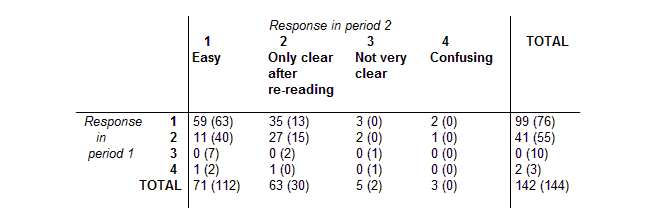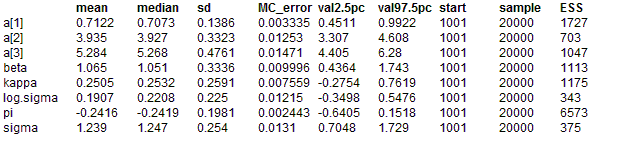#### Inhaler: ordered categorical data

Ezzet and Whitehead (1993) analyse data from a two-treatment, two-period crossover trial to compare 2 inhalation devices for delivering the drug salbutamol in 286 asthma patients. Patients were asked to rate the clarity of leaflet instructions accompanying each device, using a 4-point ordinal scale. In the table below, the first entry in each cell (r,c) gives the number of subjects in Group 1 (who received device A in period 1 and device B in period 2) giving response r in period 1 and response c in period 2. The entry in brackets is the number of Group 2 subjects (who received the devices in reverse order) giving this response pattern.The response Rit from the i th subject (i = 1,...,286) in the t th period (t = 1,2) thus assumes integer values between 1 and 4. It may be expressed in terms of a continuous latent variable Yit taking values on (-inf, inf) as follows:

Rit = j if Yit in [aj - 1, aj), j = 1,..,4

where a0 = -inf and a4 = inf. Assuming a logistic distribution with mean μit for Yit, then the cumulative probability Qitj of subject i rating the treatment in period t as worse than category j (i.e. Prob( Yit >= aj ) is given by

logitQitj = -(aj + μsit + bi)

where bi represents the random effect for subject i. Here, μsit depends only on the period t and the sequence si = 1,2 to which patient i belongs. It is defined as

μ11 = β / 2 + π / 2

μ12 = -β / 2 - π / 2 - κ

μ21 = -β / 2 + π / 2

μ22 = β / 2 - π / 2 + κ

where β represents the treatment effect, π represents the period effect and κ represents the carryover effect. The probability of subject i giving response j in period t is thus given by pitj = Qitj - 1 - Qitj, where Qit0 = 1 and Qit4 = 0 (see also the Bones example).

The BUGS language for this model is shown below. We assume the bi's to be normally distributed with zero mean and common precision τ. The fixed effects β, π and κ are given vague normal priors, as are the unknown cut points a1, a2 and a3. We also impose order constraints on the latter using the T(,) notation in BUGS, to ensure that a1 < a2 < a3.

model
{
#
# Construct individual response data from contingency table
#
for (i in 1 : Ncum[1, 1]) {
group[i] <- 1
for (t in 1 : T) { response[i, t] <- pattern[1, t] }
}
for (i in (Ncum[1,1] + 1) : Ncum[1, 2]) {
group[i] <- 2 for (t in 1 : T) { response[i, t] <- pattern[1, t] }
}

for (k in 2 : Npattern) {
for(i in (Ncum[k - 1, 2] + 1) : Ncum[k, 1]) {
group[i] <- 1 for (t in 1 : T) { response[i, t] <- pattern[k, t] }
}
for(i in (Ncum[k, 1] + 1) : Ncum[k, 2]) {
group[i] <- 2 for (t in 1 : T) { response[i, t] <- pattern[k, t] }
}
}
#
# Model
#
for (i in 1 : N) {
for (t in 1 : T) {
for (j in 1 : Ncut) {
#
# Cumulative probability of worse response than j
#
logit(Q[i, t, j]) <- -(a[j] + mu[group[i], t] + b[i])
}
#
# Probability of response = j
#
p[i, t, 1] <- 1 - Q[i, t, 1]
for (j in 2 : Ncut) { p[i, t, j] <- Q[i, t, j - 1] - Q[i, t, j] }
p[i, t, (Ncut+1)] <- Q[i, t, Ncut]

response[i, t] ~ dcat(p[i, t, ])
}
#
# Subject (random) effects
#
b[i] ~ dnorm(0.0, tau)
}

#
# Fixed effects
#
for (g in 1 : G) {
for(t in 1 : T) {
# logistic mean for group i in period t
mu[g, t] <- beta * treat[g, t] / 2 + pi * period[g, t] / 2 + kappa * carry[g, t]
}
}
beta ~ dnorm(0, 1.0E-06)
pi ~ dnorm(0, 1.0E-06)
kappa ~ dnorm(0, 1.0E-06)

# ordered cut points for underlying continuous latent variable
a ~ dunif(-1000, a)
a ~ dunif(a, a)
a ~ dunif(a, 1000)

tau ~ dgamma(0.001, 0.001)
sigma <- sqrt(1 / tau)
log.sigma <- log(sigma)

}
Note that the data is read into BUGS in the original contigency table format to economize on space and effort. The indivdual responses for each of the 286 patients are then constructed within BUGS.

##### Data
``` list(N = 286, T = 2, G = 2, Npattern = 16, Ncut = 3,   pattern = structure(.Data =          c(1, 1, 1, 2, 1, 3, 1, 4,2, 1,2, 2,2, 3,2, 4,3, 1,3, 2,3, 3,3, 4,4, 1,4, 2,4, 3,4, 4), .Dim = c(16, 2)),Ncum = structure(.Data =          c( 59, 122,157, 170,173, 173,175, 175,186, 226,253, 268,270, 270,271, 271,271, 278,278, 280,280, 281,281, 281,282, 284,       285, 285,285, 286,286, 286), .Dim = c(16, 2)),treat = structure(.Data =          c( 1, -1, -1, 1), .Dim = c(2, 2)),period = structure(.Data =          c( 1, -1, 1, -1), .Dim = c(2, 2)),carry = structure(.Data =          c( 0, -1, 0, 1), .Dim = c(2, 2))) ```
##### Inits for chain 1
``` list(beta = 0, pi = 0, kappa = 0, a = c(2, 3, 4), tau = 1) ```

##### Inits for chain 2
``` list(beta = 1, pi = 1, kappa = 0, a = c(3, 4, 5), tau = 0.1) ```
ResultsThe estimates can be compared with those of Ezzet and Whitehead, who used the Newton-Raphson method and numerical integration to obtain maximum-likelihood estimates of the parameters. They reported β = 1.17 +/- 0.75, π = -0.23 +/- 0.20, κ = 0.21 +/- 0.49, logσ = 0.17 +/- 0.23, a1 = 0.68, a2 = 3.85, a3 = 5.10